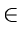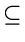Next:10.5 NP-Complete ProblemsUp:10. Introduction to NP-CompletenessPrevious:10.3.6 Satisfiability

# 10.4 The Classes P and NP

An algorithm is said to be polynomially bounded if its worst-case complexity is bounded by a polynomial function of the input size. A problem is said to be polynomially bounded if there is a polynomially bounded algorithm for it.

P is the class of all decision problems that are polynomially bounded. The implication is that a decision problem XP can be solved in polynomial time on a deterministic computation model (such as a deterministic Turing machine).

NP represents the class of decision problems which can be solved in polynomial time by a non-deterministic model of computation. That is, a decision problem XNP can be solved in polynomial-time on a non-deterministic computation model (such as a non-deterministic Turing machine). A non-deterministic model can make the right guesses on every move and race towards the solution much faster than a deterministic model.

A deterministic machine, at each point in time, executes an instruction. Depending on the outcome of executing the instruction, it then executes some next instruction, which is unique. A non-deterministic machine on the other hand has a choice of next steps. It is free to choose any that it wishes. For example, it can always choose a next step that leads to the best solution for the problem. A non-deterministic machine thus has the power of extremely good, optimal guessing.

As an example, let us consider the decision version of TSP: Given a complete, weighted graph and an integer k, does there exist a Hamiltonian cycle with total weight at most k?

A smart non-deterministic algorithm for the above problem starts with a vertex, guesses the correct edge to choose, proceeds to the next vertex, guesses the correct edge to choose there, etc. and in polynomial time discovers a Hamiltonian cycle of least cost and provides an answer to the above problem. This is the power of non-determinism. A deterministic algorithm here will have no choice but take super-polynomial time to answer the above question.

Another way of viewing the above is that given a candidate Hamiltonian cycle (call it certificate), one can verify in polynomial time whether the answer to the above question is YES or NO. Thus to check if a problem is in NP, it is enough to prove in polynomial time that any YES instance is correct. We do not have to worry about NO instances since the program always makes the right choice.

It is easy to show that PNP. However, it is unknown whether P = NP. In fact, this question is perhaps the most celebrated of all open problems in Computer Science.Next:10.5 NP-Complete ProblemsUp:10. Introduction to NP-CompletenessPrevious:10.3.6 Satisfiability
eEL,CSA_Dept,IISc,Bangalore